# How to Speed Up A* Pathfinding With the Jump Point Search AlgorithmPathfinding is ubiquitous in games. So it's important to understand the implications which are present when using algorithms such as A*. In this tutorial we're going to cover a relatively new method for searching grid based worlds: Jump Point Search, which can speed up A* by orders of magnitude.

Note: Although this tutorial is written using AS3 and Flash, you should be able to use the same techniques and concepts in almost any game development environment.

This implementation is based on the original paper and article on JPS found here: Jump Point Search. The
Lua based implementation, Jumper, was used for help with some parts of the implementation.

## Jump Point Search Demo

Click the SWF to give it focus, then move your mouse over non-blocking areas of the map to have the NPCs try to get to it. Hit Space to switch between A*, Jump Point Search, and both.

## Setup

The demo implementation above uses AS3 and Flash with the Starling Framework for GPU accelerated rendering and the polygonal-ds library for data structures.

## Pathfinding

Pathfinding is often used in video games and you are sure to bump into it at some point during your game development career. Its primary use is to give intelligent looking movement behavior to artificial entities (NPCs), to avoid them bumping into things (often).

In some games the player avatar is also subject to pathfinding (strategy games, many third person RPGs and adventure games). So you might assume that the problem of pathfinding is solved, but unfortunately that's not the case; there is no silver bullet which you can use and just be done with it.

And even in big AAA games, you will still find funny things like this:

There may not be a silver bullet, but there is a bullet: the A* (A star) algorithm. In this tutorial we are going to see a brief overview of A* and how to speed it up using another algorithm, Jump Point Search.

First we need a way to represent our game world in a way that a pathfinding algorithm can use it.

## World Representations

One of the most important things to consider when thinking about pathfinding for your game is world representation. How is the data of the passable areas and obstacles organized with programming structures in memory?

The simplest representation you can use is a grid-based structure, where path nodes are organized in a grid and can be represented by a 2D array. We are going to use this representation in this tutorial. Specifically it will be an eight-way grid representation: allowing movement in straight and diagonal directions.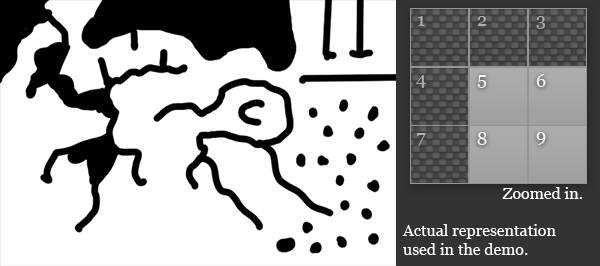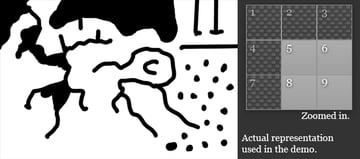The black pixels in the image represent the blocking cells.

Your requirements might be different, so this structure might not suit you. Good thing is that with some processing (usually done offline) you can change pathfinding representations to other formats. Alternatives to grid based approach would include things like polygon (obstacles represented by polygons) or navigation meshes (navigation areas represented by polygons); these can represent the same data with fewer nodes.

Another data that can be stored in map representation are costs: how much it costs to travel from one node to another. This can be used for the AI to determine the path that, for example, prefers roads over regular terrain (making the cost of the road less than the terrain).

Jump Point Search is specifically designed for eight-way grid based map representation so we'll use that. Also, in its vanilla form it doesn't support weighted maps. (In the final section I'll discuss a possible way to remedy this.)

## A* Pathfinding Refresher

Now we have a world representation let's take a quick look at implementing A*. It's a weighted graph search algorithm which uses heuristics (little "hints") of how to best search the area from the start node to the end node.

I highly recommend that you check out this visualization of pathfinding algorithms:
PathFinding.js - visual. Playing with it can boost your intuition of what the algorithm is actually doing - plus it's fun!

For pathfinding using A* in rectangular grids we do the following:

 1 2 1. Find node closest to your position and declare it start node and put it on  3  the open list.  4 2. While there are nodes in the open list:  5  3. Pick the node from the open list having the smallest F score. Put it on  6  the closed list (you don't want to consider it again).  7  4. For each neighbor (adjacent cell) which isn't in the closed list:  8  5. Set its parent to current node.  9  6. Calculate G score (distance from starting node to this neighbor) and  10  add it to the open list  11  7. Calculate F score by adding heuristics to the G value. 

Heuristics is essentially making a guess at the chance that the node being evaluated will lead to the goal. Heuristics can make a big difference in efficiency of pathfinding algorithms as they tend to limit the number of nodes that need to be visited. We are going to use Manhattan distance for our purposes (meaning that nodes closer to the goal will have a smaller number):

 1 2 private function manhattanDistance(start:Node, end:Node):int {  3  return Math.abs(end.x - start.x) + Math.abs(end.y - start.y);  4 } 

This is more or less it. We stop the algorithm when we find the goal node, and then track back using parent variable of node to construct the path.

Search algorithms can be used for other things as well. A* is a general weighted graph search algorithm, and can be used on any such graph. This can cover other fields in AI, such as finding the optimal steps to achieve certain objective: throw a bomb or run for shelter and try to sneak behind an enemy?

In game development we need to do things fast, when updating our games at 60 frames per second every millisecond counts. Even though A* performs reasonably well for some uses there exists the need to make it faster or use less memory.

## Optimizations

Choosing the representation is the first thing that will have an impact on pathfinding performance and your choice of pathfinding algorithm. The size of the graph that is being searched will have a big correlation on how your pathfinding performs (which makes sense; it's easier to find your way in your room than in a big city).

Then you would consider higher level optimizations which usually involve clustering data to smaller regions and then searching those while later refining paths in travelled smaller regions. For example, if you want to go to a restaurant in a neighboring city, you first consider how you get from your city to that one, and once you are in that city you limit your "searching" to the area where the restaurant is located, ignoring the rest. This would include things like swamps, dead end elimination and HPA*.

On the lowest level you have to do the searching. You chose your data representation and possible abstractions and then plug them in an algorithm that will pick out nodes, travel here and there searching for the goal. These algorithms are usually based on the A* searching algorithm with possible modifications. In simpler cases you can get away with using straight A* which offers you the simplicity. I've provided a grid based implementation in the source download.

## Jump Point Search

Since this tutorial is about implementing Jump Point Search, the pathfinding graph will be represented with a grid. And it specifically needs to be an eight-way grid since the algorithm directly uses it.

What Jump Point Search really does is to eliminate a lot of intermediate nodes in certain kind of grid combinations. It skips a bunch of these you would add to open list and closed list, as well as other calculations, in favor of doing some more processing when picking the next node.

As with A* we pick from the open scene the node with the lowest F score. But this is where things begin to get interesting. Instead of picking adjacent nodes we'll call this function to do it for us:

 1 2 function identifySuccessors(current:Node, start:Node, end:Node):Vector. {  3  var successors:Vector. = new Vector.();  4  var neighbours:Vector. = nodeNeighbours(current);  5   6  for each (var neighbour:Node in neighbours) {  7  // Direction from current node to neighbor:  8  var dX:int = clamp(neighbour.x - current.x, -1, 1);  9  var dY:int = clamp(neighbour.y - current.y, -1, 1);  10 11 12  // Try to find a node to jump to:  13  var jumpPoint:Node = jump(current.x, current.y, dX, dY, start, end);  14 15 16  // If found add it to the list:  17  if (jumpPoint) successors.push(jumpPoint);  18  }  19   20  return successors;  21 } 

What this does is eliminate nodes that are not interesting to our path. For this we use the direction from parent as the main guideline. Here are examples of pruning the nodes which we'll ignore for horizontal and vertical directions: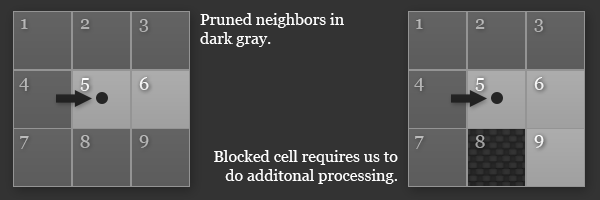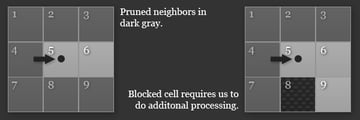Example of one of horizontal pruning situations.

In code this will end up as a series of if statements checking for these situations. You can see the example here, describing the right case from the picture:

 1 2  if(directionY == 0) {  3  if (!_world.isBlocked(current.x + directionX, current.y)) {  4  if (_world.isBlocked(current.x, current.y + 1)) {  5  // create a node with the new position  6  neighbours.push(  7  Node.pooledNode(current.x + directionX, current.y + 1));  8  }  9  }  10  }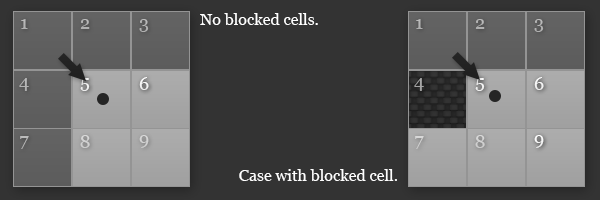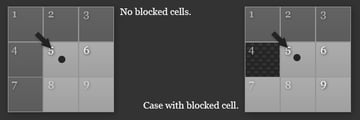Example of diagonal pruning situations.

After we pick the neighbor we try to find a jump point, which is a node which can be reached from current one, but not necessarily just in a single way. To put it more formally, what JPS does is to eliminate symmetry between paths - each has a different permutation of same moves: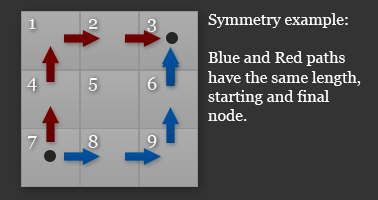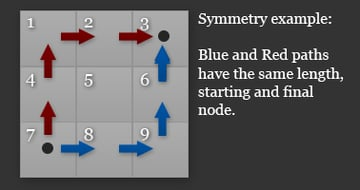Path symmetry example.

So for big open spaces we can have huge wins. Here is how the jump method works:

 1 2 function jump(cX:int, cY:int, dX:int, dY:int, start:Node, end:Node):Node {  3  // cX, cY - Current Node Position, dX, dY - Direction  4 5  // Position of new node we are going to consider:  6  var nextX:int = cX + dX;  7  var nextY:int = cY + dY;  8   9  // If it's blocked we can't jump here  10  if (_world.isBlocked(nextX, nextY)) return null;  11 12  // If the node is the goal return it  13  if (nextX == end.x && nextY == end.y) return new Node(nextX, nextY);  14 15  // Diagonal Case  16  if (dX != 0 && dY != 0) {  17  if (/*... Diagonal Forced Neighbor Check ...*/) {  18  return Node.pooledNode(nextX, nextY);  19  }  20   21  // Check in horizontal and vertical directions for forced neighbors  22  // This is a special case for diagonal direction  23  if (jump(nextX, nextY, dX, 0, start, end) != null ||  24  jump(nextX, nextY, 0, dY, start, end) != null)  25  {  26  return Node.pooledNode(nextX, nextY);  27  }  28  } else {  29  // Horizontal case  30  if (dX != 0) {  31  if (/*... Horizontal Forced Neighbor Check ...*/) {  32  return Node.pooledNode(nextX, nextY);  33  }  34  /// Vertical case  35  } else {  36  if (/*... Vertical Forced Neighbor Check ...*/) {  37  return Node.pooledNode(nextX, nextY);  38  }  39  }  40  }  41   42  /// If forced neighbor was not found try next jump point  43  return jump(nextX, nextY, dX, dY, start, end);  44 } 

I've removed the forced neighbor checks from the if statements as they are quite large. They basically consist of the checks that are similar to those when we first picked neighbors for a new node (lots of checks to see if cells are blocked). They serve the purpose of detecting when we are allowed to have our assumptions on symmetry.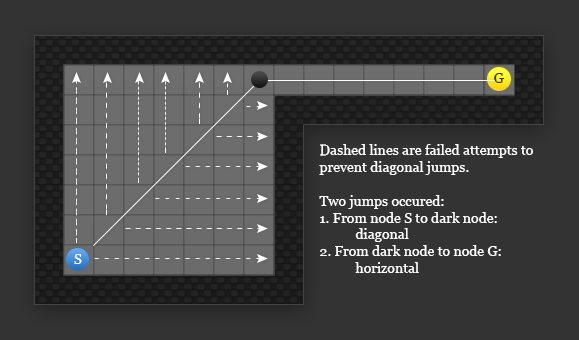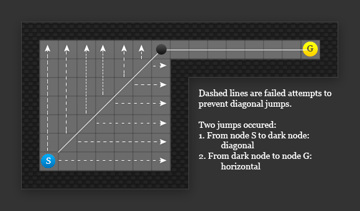Example of jump function behavior.

The diagonal case is special and we have to look not just for forced neighbors in the diagonal directions, but in the horizontal and vertical directions as well, and if any of those fail we have to put a forced node as a jump point. We also have to consider a special case of the goal node, where the jump method terminates.

Every time we don't find a search node we call the jump function recursively in the specified direction. In the demo I've actually unrolled this recursive call since this will be called a lot. (In my testing, this improved performance by a factor of two.)

This is what JPS does; the final result is new nodes for A* to check and we proceed with the algorithm. When goal node is found we reconstruct the path and return it.

## Properties

JPS can skip a lot of nodes when searching, which can give nice speed improvements (in my project it's around 30x over A*), but it does come with a cost.

It works best on a uniform grid, but can be made to work with non uniforms using additional tweaking, marking neighbors as forced where there is a transition to a node of different costs (best to use discrete costs).

In a game I'm working on, the grid is uniform except for roads, which cost much less than walking on regular terrain. (It looks much better when the character respects that.) In the end I've solved this by precomputing some values of road positions. When pathfinding is initiated, the algorithm searches possible closest points to the path from the start and goal nodes, and then searches a special high level graph of roads (which is precomputed), and then it uses JPS to search terrain areas.

## Debugging

A quick note about debugging. Debugging these kinds of algorithms can be very difficult, and it's almost certain that the first implementation will have some hard to find bug. You can do yourself a favor and build some kind of visualization functionally and draw what is happening when algorithm runs.

If you get a bug you should reduce the domain (grid size) to the minimum that lets you reproduce your problem and test from there. As you can probably guess, my first implementation of JPS did't work straight away!